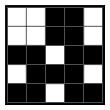﻿ Fill-a-Pix techniques
 Log inBecome a member# Fill-a-Pix techniques

Fill-a-Pix puzzles consist of a grid with clues in various places. Each clue shows how many of the nine squares - the one with the clue plus the eight around it - should be painted. When the puzzle is completed, a hidden pixel-art picture is revealed. Fill-a-Pix puzzles come in two levels, Basic Logic and Advanced Logic. Basic Logic puzzles require analysis of one clue at a time and are straightforward to solve while Advanced Logic puzzles contain additional situations where two clues simultaneously affect each other as well as the squares around them making them very challenging and rewarding to solve.

# Basic Logic Fill-a-Pix step by step solution

Basic Logic Fill-a-Pix puzzles are solved by analyzing the clues, one at a time, and painting the squares or marking them with an X according to the rules. The best way to learn how to solve Basic Logic Fill-a-Pix is to see a puzzle solved step by step from beginning to end.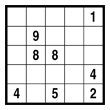## Step 1

The first step in solving Basic Logic Fill-a-Pix is to identify the starting clues - 0 and 9 inside the grid, 6 along the edges and 4 in the corners. The example below shows a highlighted area with 9 in its center. This means all nine squares - the one containing 9 and the eight around it - must be painted.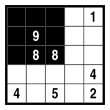## Step 2

A similar situation occurs with 4 in a corner. Since a clue in the corner of a puzzle has only three neighbors, all four squares in the highlighted area – the one containing the 4 and the three around it - must be painted.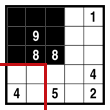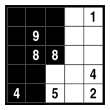## Step 3

Let’s examine the highlighted area in the next step below. The clue in the center is 8 and there are already eight squares painted from the previous steps. The logical deduction is straightforward - the bottom-right square in the highlighted area must be empty and is therefore marked with X.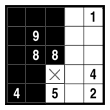## Step 4

Now let’s look at the 8 in the center of the puzzle. Since it already has one neighboring square with an X from the previous step, the three remaining squares inside the highlighted area must be painted.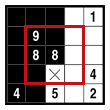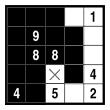## Step 5

We now move to the 5 on the bottom edge of the puzzle. The highlighted area around it consists of six squares - the square with the 5 and the five squares around it. Since the highlighted area already contains a square with an X, the two remaining empty squares must be painted.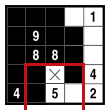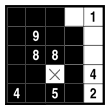## Step 6

Similarly, the 1 in the top-right corner already has a painted square as its neighbor and therefore the three remaining empty squares inside the highlighted area must be marked with X.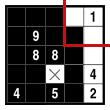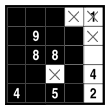## Step 7

The bottom-right corner of the puzzle contains a 2. Since two squares have been painted in previous steps, the two remaining squares in the highlighted area must be empty and are marked with X.## Step 8

By now we have solved most of the puzzle and the only remaining clue is the 4 on the right edge. As we see, two squares have been marked with X in previous steps so the last remaining empty square in the highlighted area must be painted.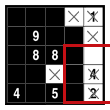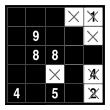## Step 9

The Basic Logic Fill-a-Pix puzzle is now complete, revealing the picture of a house.# Advanced Logic Fill-a-Pix step by step solution

Unlike Basic Logic, Advanced Logic Fill-a-Pix puzzles contain additional situations where two clues simultaneously affect each other as well as the squares around them. The best way to learn how to solve Advanced Logic Fill-a-Pix is to see a puzzle solved step by step from beginning to end.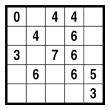## Step 1

The first step in solving Advanced Logic Fill-a-Pix is to identify the starting clues - 0 and 9 inside the grid, 6 along the edges and 4 in the corners. In this puzzle we have 0 in the top-left corner so all four squares in the highlighted area – the one containing 0 and the three around it – are empty and must be marked with X.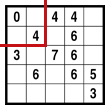## Step 2

We now continue by solving the Basic Logic situations. Let’s look at the 4 in the center-top edge of the puzzle. The highlighted area consists of six squares - the square with the 4 and the five squares around it. Since this area already contains two squares with X, the four remaining empty squares must be painted.## Step 3

This step is similar to the previous one except the highlighted area around the 4 already contains four painted squares. This means the remaining two squares are empty and must be marked with X.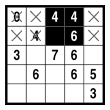## Step 4

We now have a case of Advanced Logic. Let's look first at the 3 in the bottom-right corner of the puzzle. The highlighted area around it consists of four squares, three of which must be painted. Now let’s look at the 5 in the red circle. Since there are already three painted squares belonging to it inside the highlighted area, the two additional painted squares must be the ones above the highlighted area as shown below.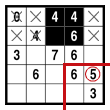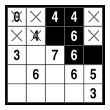## Step 5

We now continue with the 6 in the center of the highlighted area below. Since there are already six painted squares in this area, the remaining square must be empty and is marked with X.## Step 6

The 7 in the center of the puzzle is our next Basic Logic move. Since the highlighted area already contains two squares with X from previous steps, the four remaining empty squares must be painted.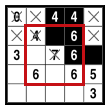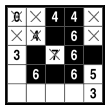## Step 7

The next step is similar to step 5, where the remaining square is empty and must be marked with X.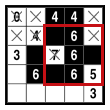## Step 8

We now move to the 5 on the bottom-right edge of the puzzle. The highlighted area around the 5 consists of six squares - the square with the 5 and the five squares around it. Since the highlighted area already contains a square with X, the two remaining empty squares must be painted.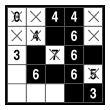## Step 9

This is another step similar to step 5, where the remaining square is empty and must be marked with X.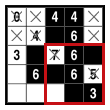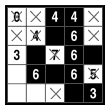## Step 10

We are now getting close to the end of the puzzle. With what we have learned so far it is immediately visible the empty square in the highlighted area below must be painted.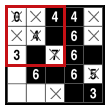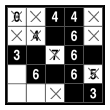## Step 11

Continuing on the left side of the puzzle, the last square in the highlighted area around the 3 is empty and must be marked with X.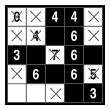## Step 12

And finally, the 6 in the highlighted area already contains three squares with X so the two remaining empty squares must be painted.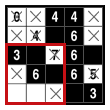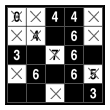## Step 13

The Advanced Logic Fill-a-Pix puzzle is now complete, revealing the picture of a boy.